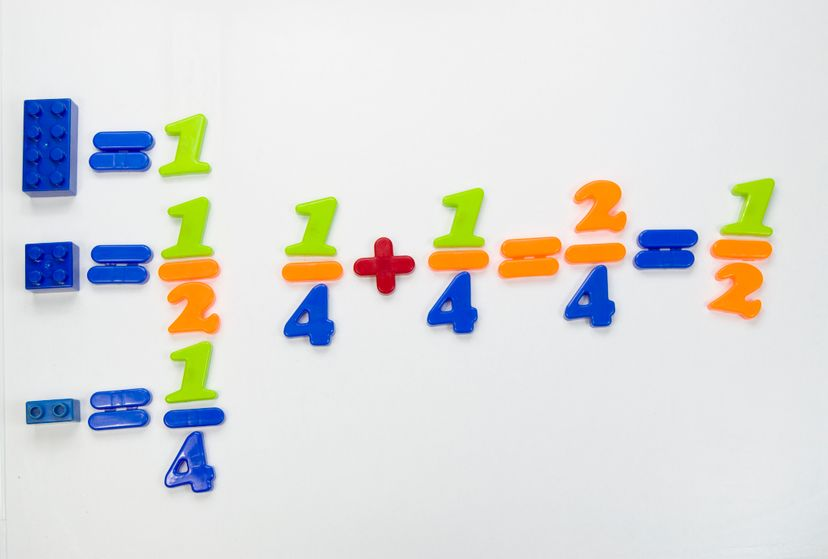# How to Add Fractions: A Step-by-Step ProcessAdding fractions is easy, once you understand how to find the least common denominator. Luis Diaz Devesa / Getty Images

If you're already familiar with subtracting fractions, learning how to add fractions will be a piece of cake for you. And if you haven't learned how to subtract fractions yet, don't worry — we've got you covered!

Contents

## What Are Fractions?

Fractions represent numbers that are not integers. Every fraction sits between two integer neighbors. Any integer can be represented as a fraction, but we like to simplify fractions into integers when possible.

For instance, you can have four halves of pie, but it's much simpler to say you have two pies. Because of this, we generally think of fractions as quotients of integers that can't be simplified into a single integer.

A fraction can be represented by dividing one integer by another: one number on top of another (a numerator on top of a denominator), separated by a short horizontal line.

## Adding Fractions With a Common Denominator

When adding fractions together, it's first important to notice whether the fractions' bottom numbers, or denominators, are the same or different.

Adding numbers with the same number for the denominator couldn't be simpler: You just add the numerators — the numbers above the horizontal line. The denominator of your answer will be the same as that of both the fractions you're adding together.

2/5 + 1/5 = 3/5

## Simplifying Fractions: Examples

When the answer's numerator and denominator share a common factor, it's standard practice to simplify the fraction. Here are two examples:

### Example A

1/4 + 1/4 = 2/4

Here, 2 and 4 share a common factor of 2, meaning you can evenly divide both of those numbers by that factor. Since 2 ÷ 2 = 1 and 4 ÷ 2 = 2, you can simplify 2/4 to 1/2.

### Example B

5/6 + 5/6 = 10/6

In this case, 10/6 is an improper fraction, meaning the numerator is larger than the denominator. Even if you divide both the numerator and denominator by a common factor of 2, the remaining fraction is 5/3.

Since 3/3 = 1, you can separate those 3 thirds from your total of 5 thirds, leaving you with 2 remaining thirds. That makes your final answer 1 2/3, which is a mixed number, because it includes both a whole number and a fraction.

## Adding Fractions With Different Denominators

### Step 1: Find the Least Common Denominator

When the denominators of the two fractions you're adding together are different (meaning they're unlike fractions), your first job is to make all the denominators the same. You'll do this by finding a common multiple of the two denominators — by convention, you find the smallest multiple. That number is called the least common denominator (LCD).

Let's find out how to find the LCD when we add these two fractions:

2/3 + 1/4

The denominator of the first fraction is 3 and that of the second fraction is 4, and both fractions are in their simplest forms. If you can't divide 3 into 4 or vice versa, you'll find the LCD by multiplying the two denominators together. In the case of the denominators 3 and 4, the LCD is the product of those two numbers: 3 x 4 = 12

### Step 2: Multiply Each Fraction By 1 to Find Equivalent Fractions

Fun fact: It's okay to multiply each term in an addition problem by 1, because anything multiplied by 1 is just itself. So, 2/2 = 1, just like 47/47 = 1.

The way to even out the denominators in an addition problem is to replace 1 with the number it will take to get the denominator of that fraction to the LCD, divided by itself.

2/3 + 1/4

(1 x 2/3) + (1 x 1/4)

For each fraction in the addition problem, you want to find out what you could multiply the denominator by in order to get the LCD. For the first fraction, that number will be 4. We then replace the 1 that we will multiply by fraction A with 4/4. The number we'd multiply the denominator by in the second fraction is 3, so we replace 1 with 3/3.

Now our expression looks like this:

(4/4 x 2/3) + (3/3 x 1/4)

Now we multiply the top and bottom numbers in both sets of fractions:

(4/4 x 2/3 = 8/12) + (3/3 x 1/4 =3/12)

From here, we add the two fractions together as normal, because each one has a new numerator and the same denominator.

8/12 + 3/12 = 11/12

### Side Note

On the other hand, if you can divide one denominator evenly into the other, then you only need to convert one fraction, not both. For instance, if we were instead adding 1/3 + 5/6, the first fraction's denominator (3) divides evenly into the second one's denominator (6).